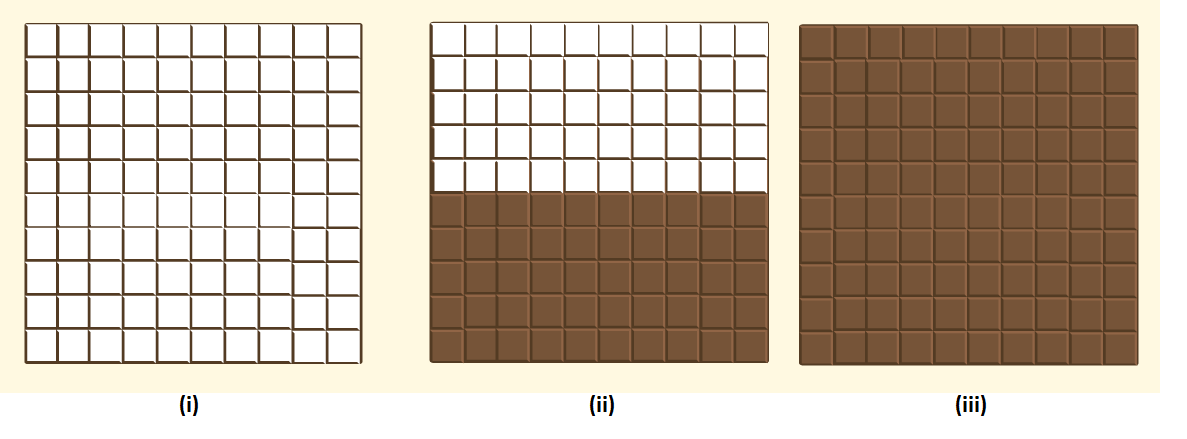### Percentage

In mathematics, a percentage is a number or ratio that can be expressed as a fraction of 100, which means, a part per hundred. The word per cent means per 100. It represented by the symbol “%”. Percentages have no dimension. Hence it is called a dimensionless number. If we say, 50% of a number, then it means 50 percent of its whole. Also, learn

Percentages can also be represented in decimal or fraction form, such as 0.6%, 0.25%, etc. In academics, the marks obtained in any subject are calculated in terms of percentage. Like, Ram has got 78% of marks in his final exam. So, this percentage is calculated on account of total marks obtained by Ram in all subjects to the total marks.## Percentage Formula

To determine the percentage, we have to divide the value by the total value and then multiply the resultant to 100.

Percentage formula = (Value/Total value)×100

Example: 2/5 × 100 = 0.4 × 100 = 40 per cent

### How to calculate the percentage of a number?

To calculate the percentage of a number, we need to use a different formula such as:

P% of Number = X

where X is the required percentage.

If we remove the % sign, then we need to express the above formulas as;

P/100 * Number = X

Example: Calculate 10% of 80.

Let 10% of 80 = X

10/100 * 80 = X

X = 8

## Percentage Example

Two quantities are generally expressed on the basis of their ratios. Here, let us understand the concepts of percentage through a few examples in a much better way.

 Examples: Let a bag contain 2 kg of apples and 3kg of grapes. Find the ratio of quantities present, and percentage occupied by each.Solution: The number of apples and grapes in a bag can be compared in terms of their ratio, i.e. 2:3.The actual interpretation of percentages can be understood by the following way:The same quantity can be represented in terms of percentage occupied, which is given as:Total quantity present = 5 kgRatio of apples (in terms of total quantity) = 25= 25×100100From the definition of percentage, it is the ratio that is expressed per hundred,1100=1%Thus, Percentage of Apples = 25×100=40Percentage of Grapes = 35×100=60

## Converting Fractions to Percentage

A fraction can be represented by ab.

Multiplying and dividing the fraction by 100, we have

ab×100100

=(ab×100)1100 ………………(i)

From the definition of percentage, we have =1100 = 1%

Thus equation (i) can be written as:

=ab×100%

Thus fraction can be converted to percentage simply by multiplying the given fraction by 100.

## Difference between Percentage and Percent

The word percentage and percent are related closely to each other.

Percent ( or symbol %) is accompanied by a specific number.

E.g., More than 75% of the participants responded with their positive response to abjure.

The percentage is represented without a number.

E.g., The percentage of the population affected by malaria is between 60% and 65%.

Fractions, Ratios, Percents and Decimals are interrelated with each other. Let us look on to the conversion of one form to other:

 S.no Ratio Fraction Percent(%) Decimal 1 1:1 1/1 100 1 2 1:2 1/2 50 0.5 3 1:3 1/3 33.333 0.3333 4 1:4 1/4 25 0.25 5 1:5 1/5 20 0.20 6 1:6 1/6 16.667 0.16667 7 1:7 1/7 14.285 0.14285 8 1:8 1/8 12.5 0.125 9 1:9 1/9 11.111 0.11111 10 1:10 1/10 10 0.10 11 1:11 1/11 9.0909 0.0909 12 1:12 1/12 8.333 0.08333 13 1:13 1/13 7.692 0.07692 14 1:14 1/14 7.142 0.07142 15 1:15 1/15 6.66 0.0666

## Percentage in Maths

Every percentage problem has three possible unknowns or variables :

• Percentage
• Part
• Base

In order to solve any percentage problem, you must be able to identify these variables.

Look at the following examples. All three variables are known:

Example: 70% of 30 is 21

70 is the percentage.

30 is the base.

21 is the part.

Example: 25% of 200 is 50

25 is the percent.

200 is the base.

50 is the part.

Example: 6 is 50% of 12

6 is the part.

50 is the percent.

12 is the base.

## Percentage Tricks

To calculate the percentage, we can use the given below tricks.

 x % of y = y % of x
 Example- Prove that 10% of 30 is equal to 30% of 10.Solution- 10% of 30 = 330% of 10 = 3Therefore they are equal i.e. x % of y = y % of x holds true.

## Problems on Percentage

 Example- Suman has a monthly salary of $1200. She spends$280 per month on food. What percent of her monthly salary does she save?Solution- Suman’s monthly salary = $1200Savings of Suman =$(1200 – 280) = \$ 920Fraction of salary she saves = 9201200Percentage of salary she saves = 9201200×100=92012=76.667 %Example- Below given are three grids of chocolate. What percent of each White chocolate bar has Dark chocolate bar?Solution- Each grid above has 100 white chocolate blocks. For each white chocolate bar, the ratio of the number of dark chocolate boxes to the total number of white chocolate bars can be represented as a fraction.(i) 0 dark and 100 white.i.e. 0 per 100 or 0%.(ii) 50 dark and 50 white.I.e. 50 per 100 or 50%.(iii) 100 dark and 0 white.I .e., 100 per 100 or 100%.